# File list

This special page shows all uploaded files.

File list
 Items per page: 20 50 100 250 500 Search for media name: Username: Include old versions of images
Date Name Thumbnail Size Description Versions
01:09, 23 November 2010 Upperblockforcediagram.png (file)2 KB This file was created using [http://www.wolfram.com/mathematica Wolfram Mathematica 7] with the following code: <pre>Graphics[{Line[{{0.2, 1}, {1.8, 1}, {1.8, 2}, {0.2, 2}, {0.2, 1}}], PointSize[0.02], Point[{1, 1.5}], Arrow[{{1, 1.5}, {1, 0}}], Te 1
00:57, 23 November 2010 Pileoftwoblocks.png (file)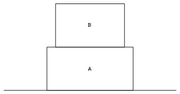1 KB This file was created using [http://www.wolfram.com/mathematica Wolfram Mathematica 7] using the following code: <pre>Graphics[{Line[{{-1, 0}, {3, 0}}], Line[{{0, 0}, {2, 0}, {2, 1}, {0, 1}, {0, 0}}], Line[{{0.2, 1}, {1.8, 1}, {1.8, 2}, {0.2, 2}, { 1
22:49, 22 November 2010 Fixedpulleywithverticalloads.png (file)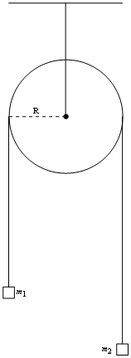5 KB This file was created using [http://www.wolfram.com/mathematica Wolfram Mathematica 7] with the following code: <pre>Graphics[{Circle[{0, 0}, 1], Line[{{0, 2}, {0, 0}}], PointSize[0.04], Point[{0, 0}], Line[{{-1, 2}, {1, 2}}], Line[{{-1, 0}, {-1, -3}} 1
22:27, 22 November 2010 Blockoncircularincline.png (file)9 KB This file was created using [http://www.wolfram.com/mathematica Wolfram Mathematica] using the following code: <pre>Graphics[{Brown, Circle[{0, 0}, Sqrt], Black, Line[{{1.1, 0.9}, {0.9, 1.1}, {1.1, 1.3}, {1.3, 1.1}, {1.1, 0.9}}], PointSize[0.02] 1
00:07, 18 September 2010 Blockonflatsurface.png (file)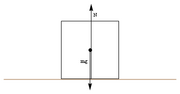2 KB This file was created using [http://www.wolfram.com/mathematica Wolfram Mathematica 7] with the following command: <pre>Graphics[{White, EdgeForm[Black], Polygon[{{0, 0}, {1, 0}, {1, 1}, {0, 1}}], Black, PointSize[0.02], Point[{0.5, 0.5}], Brown, L 1
23:19, 17 September 2010 Topplableblockonincline.png (file)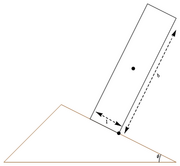6 KB This file was created using [http://www.wolfram.com/mathematica Wolfram Mathematica 7] with the following command: <pre>Graphics[{White, EdgeForm[Brown], Polygon[{{0, 0}, {3, 0}, {1, 1}}], EdgeForm[Black], Polygon[{{1.5, 0.75}, {2, 0.5}, {3, 2.5}, 1
21:43, 17 September 2010 Adjacentblocksonincline.png (file)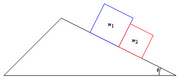3 KB This file was created using [http://www.wolfram.com/mathematica Wolfram Mathematica 7] with the following command: <pre>Graphics[{White, EdgeForm[Black], Polygon[{{0, 0}, {3, 0}, {1, 1}}], EdgeForm[Blue], Polygon[{{1.5, 0.75}, {2, 0.5}, {2.25, 1}, 1
21:30, 17 September 2010 Blockoninclinenoslidingforcediagram.png (file)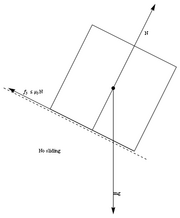7 KB   2
21:26, 17 September 2010 Blockoninclineslidingupforcediagram.png (file)7 KB   2
21:25, 17 September 2010 Blockoninclineforcediagramnormalcomponents.png (file)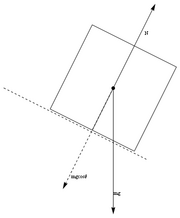8 KB This file was created using [http://www.wolfram.com/mathematica Wolfram Mathematica 7] with the following command: <pre>Graphics[{White, EdgeForm[Black], Polygon[{{0, 3}, {2, 2}, {3, 4}, {1, 5}}], Black, PointSize[0.02], Point[{1.5, 3.5}], Arrow[{{ 1
21:07, 17 September 2010 Blockoninclineslidingdownforcediagram.png (file)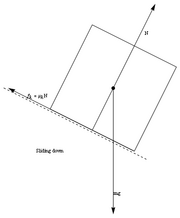7 KB This file was created using [http://www.wolfram.com/mathematica Wolfram Mathematica 7] with the following command: <pre>Graphics[{White, EdgeForm[Black], Polygon[{{0, 3}, {2, 2}, {3, 4}, {1, 5}}], Black, PointSize[0.02], Point[{1.5, 3.5}], Arrow[{{ 1
20:30, 17 September 2010 Blockonwedge.png (file)2 KB This file was created using [http://www.wolfram.com/mathematica Wolfram Mathematica 7] with the following command: <pre>Graphics[{White, EdgeForm[Brown], Polygon[{{0, 0}, {3, 0}, {1, 1}}], EdgeForm[Black], Polygon[{{1.5, 0.75}, {2, 0.5}, {2.25, 1}, 1
01:13, 17 September 2010 Pulleysystemondoubleinclinedplane.png (file)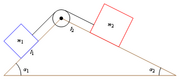4 KB This file was created using [http://www.wolfram.com/mathematica Wolfram Mathematica 7] with the following command: <pre>Graphics[{White, EdgeForm[Brown], Polygon[{{0, 0}, {3, 0}, {1, 1}}], White, EdgeForm[Red], Polygon[{{1.5, 0.75}, {2, 0.5}, {2.25 1
01:02, 17 September 2010 Blocksontwoinclinesoffreewedge.png (file)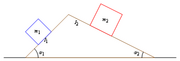3 KB   2
00:41, 17 September 2010 Angleofrollingindegrees.png (file)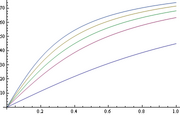13 KB This file was created using [http://www.wolfram.com/mathematica Wolfram Mathematica 7] using the following command: <pre>Plot[{ArcTan[t] * 180/Pi, ArcTan[2 t] * 180/Pi, ArcTan[3 t] * 180/Pi, ArcTan[5 t/2] * 180/Pi, ArcTan[7 t/2] * 180/Pi}, {t, 0, 1}]< 1
00:40, 17 September 2010 Angleofrolling.png (file)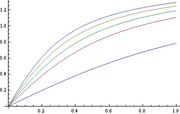13 KB This file was created using [http://www.wolfram.com/mathematica Wolfram Mathematica 7] using the following command: <pre>Plot[{ArcTan[t], ArcTan[2 t], ArcTan[3 t], ArcTan[5 t/2], ArcTan[7 t/2]}, {t, 0, 1}]</pre> 1
23:44, 16 September 2010 Rotatoronincline.png (file)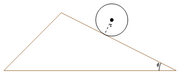4 KB This file was created using [http://www.wolfram.com/mathematica Wolfram Mathematica 7] using the following command: <pre>Graphics[{White, EdgeForm[Brown], Polygon[{{0, 0}, {3, 0}, {1, 1}}], Black, PointSize[0.02], Point[{1.875, 0.875}], Circle[{1.8 1
23:45, 15 September 2010 Upaccelerationintermsofinclineangle.png (file)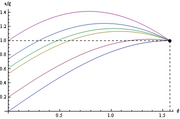13 KB   2
23:43, 15 September 2010 Downaccelerationintermsofinclineangle.png (file)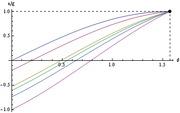13 KB   2
23:26, 15 September 2010 Mgcomponentsforincline.png (file)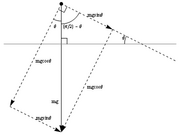7 KB   3
22:32, 15 September 2010 Angleoffrictionintermsofcoefficientindegrees.png (file)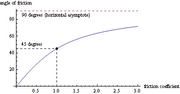4 KB   2
22:29, 15 September 2010 Commonfrictioncoefficientsandanglesoffrictionindegrees.png (file)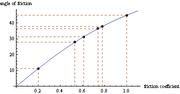5 KB This file was created using [http://www.wolfram.com/mathematica Wolfram Mathematica] using the following command: <pre>Plot[ArcTan[x] * 180/Pi, {x, 0, 1.1}, AxesOrigin -> {0, 0}, AxesLabel -> {"friction coefficient", "angle of friction"}, Epilog -> { 1
22:20, 15 September 2010 Angleoffrictionintermsofcoefficientindegrees2.png (file)4 KB This file was created using [http://www.wolfram.com/mathematica/ Wolfram Mathematica 7] using the following command: <pre>Plot[{ArcTan[x] * 180/Pi, 90}, {x, 0, 20}, PlotStyle -> {Automatic, Dashed}, AxesOrigin -> {0, 0}, AxesLabel -> {"friction coeff 1
22:14, 15 September 2010 Commonfrictioncoefficientsandanglesoffriction.png (file)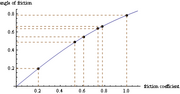5 KB Thile file was created using [http://www.wolfram.com/mathematica Wolfram Mathematica 7] with the following command: <pre>Plot[ArcTan[x], {x, 0, 1.1}, AxesOrigin -> {0, 0}, AxesLabel -> {"friction coefficient", "angle of friction"}, Epilog -> {PointSi 1
22:13, 15 September 2010 Angleoffrictionintermsofcoefficient2.png (file)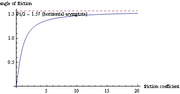4 KB This file was created using [http://www.wolfram.com/mathematica Wolfram Mathematica 7] with the following command: <pre>Plot[{ArcTan[x], Pi/2}, {x, 0, 20}, PlotStyle -> {Automatic, Dashed}, AxesOrigin -> {0, 0}, AxesLabel -> {"friction coefficient", 1
22:11, 15 September 2010 Angleoffrictionintermsofcoefficient.png (file)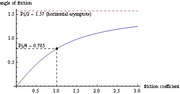4 KB This file was created using [http://www.wolfram.com/mathematica Wolfram Mathematica] with the following code: <pre>Plot[{ArcTan[x], Pi/2}, {x, 0, 3}, PlotStyle -> {Automatic, Dashed}, AxesOrigin -> {0, 0}, AxesLabel -> {"friction coefficient", "angle 1
23:34, 11 September 2010 Blockonincline.png (file)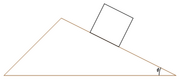2 KB This file was created using [http://www.wolfram.com/mathematica Wolfram Mathematica 7] using the following command: <pre>Graphics[{White, EdgeForm[Brown], Polygon[{{0, 0}, {3, 0}, {1, 1}}], Black, Line[{{1.5, 0.75}, {2, 0.5}}], Line[{{2, 0.5}, {2.25, 1
18:08, 26 January 2009 Logo.jpg (file)32 KB Logo of 4AM. 1